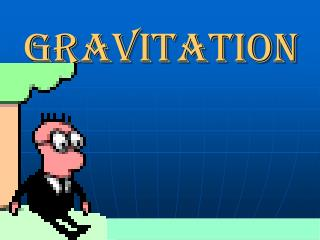DownloadDownload PresentationGRAVITATION

# GRAVITATION

Download Presentation## GRAVITATION

- - - - - - - - - - - - - - - - - - - - - - - - - - - E N D - - - - - - - - - - - - - - - - - - - - - - - - - - -
##### Presentation Transcript

1. GRAVITATION

2. NEWTON’S LAW OF GRAVITATION • There is an attractive force between any two bodies which is directly proportional to the product of their masses and inversely proportional to the square of the distance between their centers of mass.

3. Fa Fb d NEWTON’S LAW OF GRAVITATION mb ma

4. N.m2 Kg2 NEWTON’S LAW OF GRAVITATION Universal Gravitational Constant G = 6.67 X 10-11

5. Note : FGrav = Fw NEWTON’S LAW OF GRAVITATION (The weight of a body is the gravitational force acting on it) m1 FGrav = Fw re m1g = Me

6. m1 g = re Me NEWTON’S LAW OF GRAVITATION This expression can be used to find the acceleration due to gravity on any celestial body of known mass and radius.

7. NEWTON’S LAW OF GRAVITATION g = (9.80 m/s2)(6.37 X 106 m)2 Me = 6.67 X 10-11 N.m2/ Kg2 Given that the Earth’s radius Is 6.37 X 106 m, find the Earth’s mass. Me = 5.96 X 1024 Kg

8. NEWTON’S LAW OF GRAVITATION The Earth has a mass of 5.96 X 1024 Kg and the moon has a mass of 7.32 X 1022 Kg. If the moon orbits the Earth at a distance of 3.8 X 105 Km, what force does the moon exert on the Earth? d Me Mm

9. NEWTON’S LAW OF GRAVITATION me = 5.96 X 1024 Kg mm=7.32 X 1022 Kg d = 3.8 X 105 Km = 3.8 X 108 m FGrav = 2.0 X 1020 N

10. NEWTON’S LAW OF GRAVITATION How far apart would the centers of two 1000 Kg bodies have to be in order for the gravitational attraction between them to be 1.0 N ? m1 = m2 = m = 1000 Kg F m1 m2 N.m2 Kg2 G = 6.67 X 10-11 F = 1.0 N

11. Fgrav m1 m2 NEWTON’S LAW OF GRAVITATION d=8.2 X 10-3 m

12. MOVE ON TO CIRCULAR MOTION